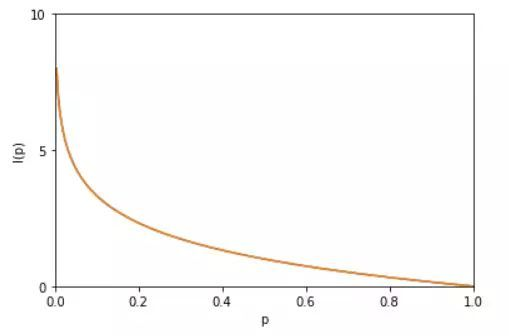## 1 信息熵

$$I(x)=−\log {p(x)}$$$$H(X)=-\sum_{x}p(x)\log p(x)=-\sum_{i=1}^{n}p(x_{i})\log p(x_{i})=-\int_x p(x)\log p(x)dx$$

$$H(X)$$ 就被称为随机变量 $$x$$ 的熵,它是表示随机变量不确定的度量是对所有可能发生的事件产生的信息量的期望

$$H(X,Y)=-\sum_{x,y} \log p(x,y) = -\sum_{i=1}^{n} \sum_{j=1}^{m} p(x_i,y_i) \log p(x_i,y_i)$$

$$H(X)=−4\times{\frac {1}{4}}\times\log_{2} {\frac {1}{4}}=2\ bits$$

$$H(X)=-\frac{1}{2} \log_2{\frac{1}{2}}-\frac{1}{4} \log_2{\frac{1}{4}}-\frac{1}{8} \log_2{\frac{1}{8}}-\frac{1}{8} \log_2{\frac{1}{8}}=1.75 \ bits$$

$$average\ code\ length= {\frac {1}{2}}\times{1} +{\frac {1}{4}}\times 2+ 2 \times {\frac {1}{8}} \times 3 = 1.75\ bits$$

### 1.1 小结

1. 熵只依赖于随机变量的分布,与随机变量取值无关；
2. 定义0log0=0(因为可能出现某个取值概率为0的情况)；
3. 熵越大,随机变量的不确定性就越大,分布越混乱，随机变量状态数越多。

### 1.2 证明 0 ≤ H(X) ≤ logn 证明 \$$0≤H(X)≤logn\$$ 利用拉格朗日乘子法证明： 因为 \$$p(1)+p(2)+⋯+p(n)=1\$$ 所以有：

$$f(p(1),p(2),…,p(n))=\{-(p(1)logp(1)+p(2)logp(2)+⋯+p(n)logp(n))\}$$

$$g(p(1),p(2),…,p(n),λ)=p(1)+p(2)+⋯+p(n)−1=0$$

$$L(p(1),p(2),…,p(n),λ)={−(p(1)logp(1)+p(2)logp(2)+⋯+p(n)logp(n))+λ(p(1)+p(2)+⋯+p(n)−1))}$$

$$λ−log(e\times p(1))=0$$ $$λ−log(e\times p(2))=0$$ $$\cdots$$ $$λ−log(e\times p(n))=0$$ $$p(1)+p(2)+⋯+p(n)−1=0$$

$$f({1 \over n},{1 \over n},\cdots ,{1 \over n})=-({1 \over n}\log{1 \over n}+{1 \over n}\log{1 \over n}+\cdots+{1 \over n}\log{1 \over n})=-\log{1 \over n}=\log n$$

## 2 条件熵

Conditional entropy

$$H(Y|X) ={ \sum_{x} p(x)H(Y|X=x)}$$ $$={-\sum_{x}p(x) \sum_{y} p(y|x) \log p(y|x)}$$ $$={-\sum_{x} \sum_{y} p(x,y) \log p(y|x)}$$ $$={-\sum_{x,y}p(x,y) \log p(y|x)}$$ $$={-\sum_{x,y}p(x,y) \log p(y|x)}$$

$$H(X,Y) ={-\sum_{x,y} p(x,y)\log p(x,y)}$$ $$={-\sum_{x,y} p(x,y) \log (p(y|x)p(x))}$$ $$={-\sum_{x,y} p(x,y) \log p(y|x)-\sum_{x,y}p(x,y) \log p(x)}$$ $$={H(Y|X)-\sum_{x,y} p(x,y) \log p(x)}$$ $$= {H(Y|X)-\sum_{x} \sum_{y} p(x,y) \log p(x)}$$ $$={H(Y|X)-\sum_{x} \log p(x) \sum_{y} p(x,y)}$$ $$={H(Y|X)-\sum_{x} \log p(x) p(x)}$$ $$= {H(Y|X)-\sum_{x} p(x)\log p(x)}$$ $$={H(Y|X)+H(X)}$$

## 3 相对熵 / KL散度

$$D_{KL}(p||q)=\sum_{x}p(x)\log {\frac {p(x)}{q(x)}}=\sum_{x}p(x)(\log p(x)-\log q(x))=E_{p(x)}\log {\frac {p(x)}{q(x)}}$$

K-L散度度量信息损失：

$$\sum_{x}p(x)(\log p(x)-\log q(x))$$

### 3.1 性质

• 如果 $$p(x)$$ 和 $$q(x)$$ 两个分布相同，那么相对熵等于0
• 非对称性： $$D_{KL} (p||q) ≠ D_{KL}(q||p)$$，相对熵具有不对称性。大家可以举个简单例子算一下。
• 非负性：$$D_{KL}(p||q)≥0$$

Cross entropy